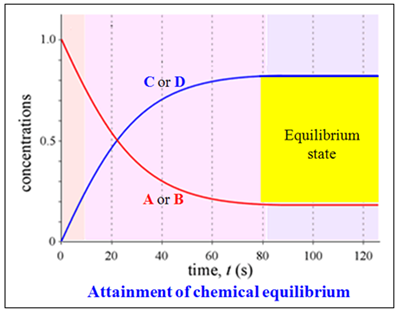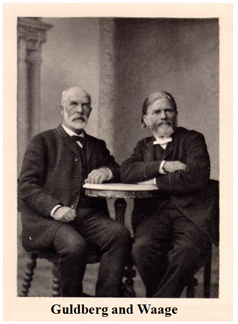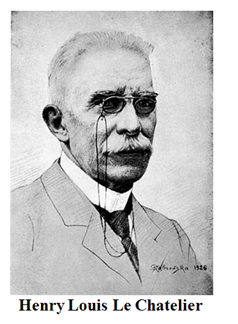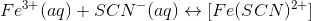Chemical Equilibrium

# Our Objective

Our objective is to study the shift of equilibrium between ferric ions and thiocyanate ions by increasing the concentration of either of them.

# TheoryLet's see how chemical equilibria behave when concentration changes.

Many chemical reactions involve a state of equilibrium. Equilibrium is said to be dynamic when the rate of forward reaction become equal to that of the backward reaction.

Consider a general reversible reaction;With the passage of time, there is a depletion of the reactants A and B and an accumulation of the products C and D. As a result, the rate of forward reaction decreases and that of backward reaction increases. Eventually, the two reactions occur at the same rate and the system reaches a state of equilibrium.

## Let us explain the 'Law of Chemical Equilibrium'

Norwegian chemists Cato Maxmillian Guldberg and Peter Waage proposed that for a chemical reaction;The rate of reaction in either direction is proportional to the active mass (concentration) of the reactants.

By applying the Law of Mass Action;

The rate of forward reaction;The rate of back ward reaction;Where [A], [B], [C] and [D] are the equilibrium concentrations of A, B, C and D respectively.

a, b, c, and d represents the stoichiometric coefficients of A, B, C and D respectively.

Kf  and K are the rate constants of forward and backward reaction.

However, at equilibrium,

Rate of forward reaction = Rate of backward reaction.or,or,where,Kc is called the equilibrium constant expressed in terms of molar concentrations and the above equation is known as the Law of Chemical Equilibrium.

## What does the Law of Chemical Equilibrium state?

The Law of Chemical Equilibrium is defined as, the ratio of product of concentration of the products to the product of concentration of the reactants, with each concentration term is raised to the power by its coefficient in overall balanced chemical equation, is a constant quantity at a given temperature and it is called equilibrium constant.## What then is "Le Chatelier’s Principle", and how does it to apply it to reactions in a state of dynamic equilibrium?

If a system is subjected to a change in concentration of one or more reactants, or a change in temperature or pressure, the equilibrium condition of the system is altered. A net reaction will take place in some direction until a new equilibrium state is attained.

In 1884, a French Chemist and Engineer, Le Chatelier, showed that in every such case, the new equilibrium state partially reduces the effect of change that brought it about. This principle is known as Le Chatelier’s Principle.

The principle states that, if a system at equilibrium is subjected to a change of pressure or temperature or number of moles of the component, there will be a tendency for a net reaction in the direction that reduces the effect of this change.

## Effect of concentration change

According to Le Chatelier’s principle, when the concentration of any of the reactants or products in a reaction at equilibrium is changed, the composition of the equilibrium mixture changes so as to minimize the effect of concentration changes.

To illustrate the effect of change in concentration on equilibrium, consider the equilibrium between ferric ions and thiocyanate ions;The equilibrium constant for the above reaction can be written as,Where, [Fe(SCN)2+], [ Fe3+] and [ SCN-] are the equilibrium concentrations of the respective species and Kc is the equilibrium constant.

[Fe(SCN)2+]/[ Fe3+][ SCN-] is known as the reaction quotient as is denoted by Qc. It can be expressed in the same way as equilibrium constant Kc except that the concentrationsin Qc are not necessarily the equilibrium values.

In this reaction, ferric chloride reacts with potassium thiocyanate to form a deep red-coloured ferric thiocyanate complex. The intensity of the red colour becomes constant on attaining equilibrium.

When the concentration of any of these species is changed, the equilibrium is disturbed and the reaction quotient remains no longer equal to equilibrium value. In order to re-establish the equilibrium, the ions interact in such a way that the reaction quotient again becomes equal to the equilibrium constant.

• If we add ferric chloride solution to the deep red-coloured solution containing ferric ions, thiocyanate ions and ferric thiocyanate complex, the concentration of ferric ions increases. As a result, more thiocyanate ions combine with ferric ions to increase the [Fe(SCN)2+] complex and therefore the colour intensity of the red solution increases. The increase in concentration of ferric ions shifts the equilibrium in the forward direction.
• If we increase the concentration of thiocyanate, more ferric ions react with thiocyanate ions to increase the [Fe(SCN)2+] complex. As a result, the colour intensity of the red solution increases. Thus, the increase in concentration of thiocyanate ions shifts the equilibrium in the forward direction.
• If we add potassium chloride to the solution, the concentration of potassium ions increases.It affects the equilibrium between potassium ions and thiocyanate ions.As the concentration of potassium ions increases, more thiocyanate ions react with potassium ions to form more potassium thiocyanate and the above equilibrium shift in the backward direction occurs. As a result, the concentration of thiocyanate ions decreases and the equilibrium 1 shifts in the backward direction. In other words, some of the [Fe(SCN)2+] complex dissociates to give  Fe3+ and  SCN-  ions.

As a result, the concentration of [Fe(SCN)2+] decreases and therefore the intensity of red colour decreases. Thus the increase in concentration of K+ ions shifts the equilibrium in the backward direction.

Generally we can say that;

• An increase in the concentration of the reactants shifts the equilibrium in the forward direction and a decrease in the  concentration of the reactants shifts the equilibrium in the backward direction.
• Similarly, an increase in concentration of the products shifts the equilibrium in the backward direction and a decrease in  the concentration of the products shifts the equilibrium in the forward direction.

# Learning outcomes

• Students understand the term ‘chemical equilibrium’ through the experiment.
• Students understand the effect of change in concentration on the equilibrium of a reaction.
• Students understand the shift in equilibrium position with respect to the concentration change.
• Students are better able to perform the experiment in the real lab after experiencing it in the virtual lab.

Cite this Simulator: